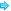科研进展新闻动态科研进展综合新闻传媒扫描
 现在位置：首页 > 新闻动态 > 科研进展

2023-05-25 | 编辑：

We consider half-harmonic maps from \mathbb{R} (or \mathbb{S}) to \mathbb{S}. We prove that all (finite energy) half-harmonic maps are non-degenerate. In other words, they are integrable critical points of the energy functional. A full description of the kernel of the linearized operator around each half-harmonic map is given. The second part of this paper devotes to studying the quantitative stability of half-harmonic maps. When its degree is \pm 1, we prove that the deviation of any map \boldsymbol{u}:\mathbb{R}\to \mathbb{S} from M？bius transformations can be controlled uniformly by \|\boldsymbol{u}\|_{\dot H^{1/2}(\mathbb{R})}^2-deg \boldsymbol{u}. This result resembles the quantitative rigidity estimate of degree \pm 1 harmonic maps \mathbb{R}^2\to \mathbb{S}^2 which is proved recently. Furthermore, we address the quantitative stability for half-harmonic maps of higher degree. We prove that if \boldsymbol{u} is already near to a Blaschke product, then the deviation of \boldsymbol{u} to Blaschke products can be controlled by \|\boldsymbol{u}\|_{\dot H^{1/2}(\mathbb{R})}^2-deg \boldsymbol{u}. Additionally, a striking example is given to show that such quantitative estimate can not be true uniformly for all \boldsymbol{u} of degree 2. We conjecture similar things happen for harmonic maps {\mathbb R}^2\to {\mathbb S}^2.

Publication:

Advances in Mathematics, Volume 420, 1 May 2023, 108979

Author:

Bin Deng

Department of Mathematics and Statistics, Wuhan University, Wuhan, Hubei Province, 430072, PR China

Liming Sun

Academy of Mathematics and Systems Science, the Chinese Academy of Sciences, Beijing 100190, PR China

Email: lmsun@amss.ac.cn

Jun-cheng Wei

Department of Mathematics, University of British Columbia, Vancouver, BC, V6T 1Z2, Canada

【打印本页】【关闭本页】